# Longest Bitonic Subsequence | (DP-46)

Problem Statement:

Longest Bitonic Subsequence

Given an array, ‘Arr’ of length ‘n’, find the longest bitonic subsequence.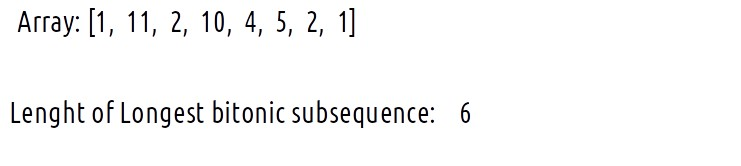Problem Statement:

Let us first understand what a bitonic subsequence means.

A bitonic subsequence is a subsequence of an array in which the elements can be any of these three:

• First, increase till a point and then decrease.
• Goes on increasing (Longest increasing subsequence)
• Goes on decreasing (Longest decreasing subsequence)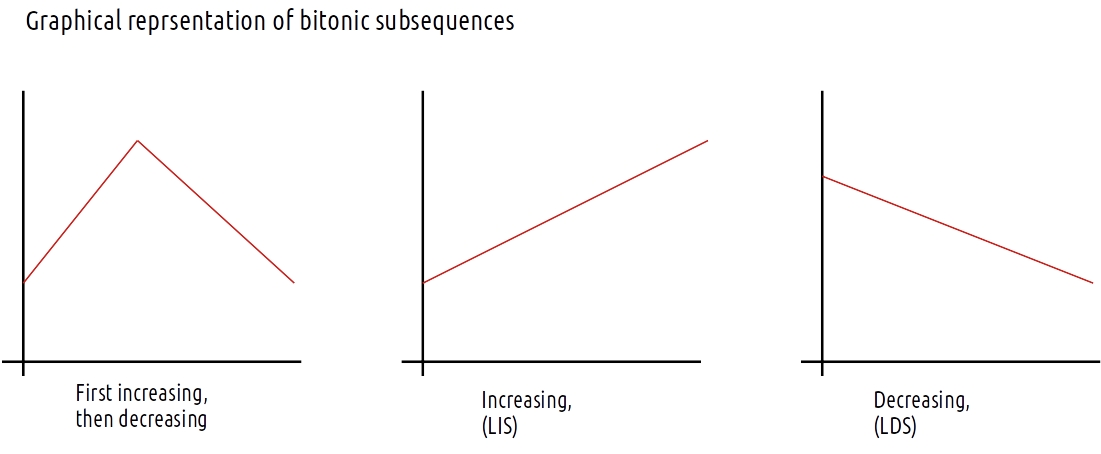Bitonic subsequence for the below example is: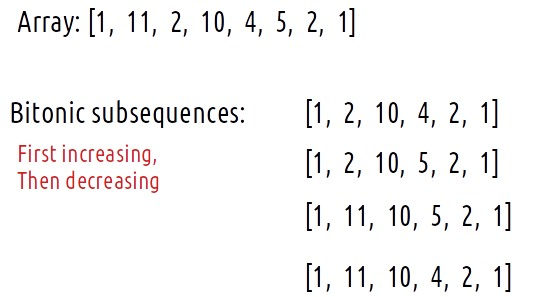Similarly, a subsequence having the elements in either increasing or decreasing order only also counts as a bitonic subsequence. For example: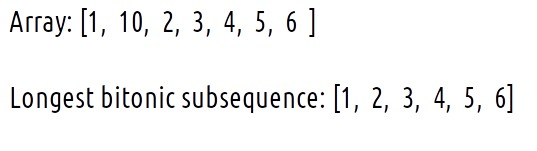We need to return the length of the longest bitonic subsequence as our answer.

### Intuition:

The length of the increasing and then decreasing subsequence gives us the hint to think in terms of the longest increasing subsequence (LIS). Now let us revise the approach for finding the LIS as discussed in Printing Longest Increasing subsequence. Readers are highly advised to understand that approach first. In that solution, we write two nested loops to get a dp array whose maximum element gives us the length of the LIS as shown below: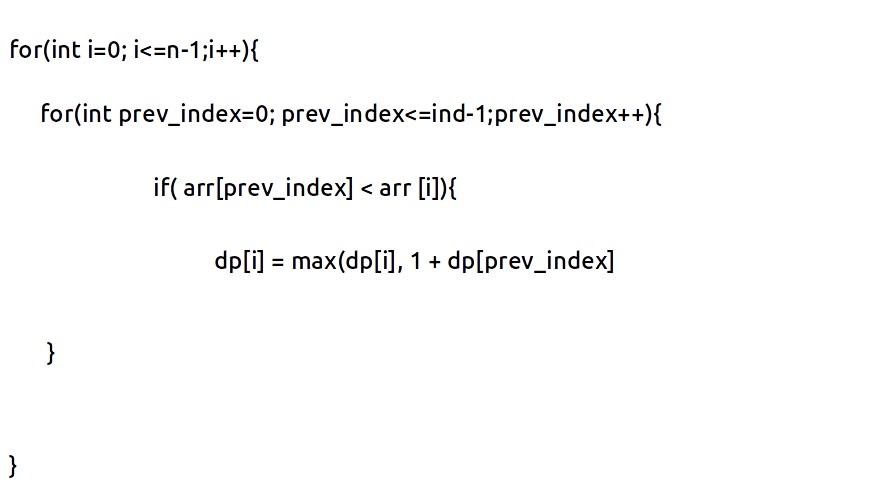Now, what does this dp[i] represent? It represents the LIS in the array ‘arr’ from index 0 to index i in the array ending with element arr[i]. Therefore the maximum value of dp[i] gives us the LIS of the array.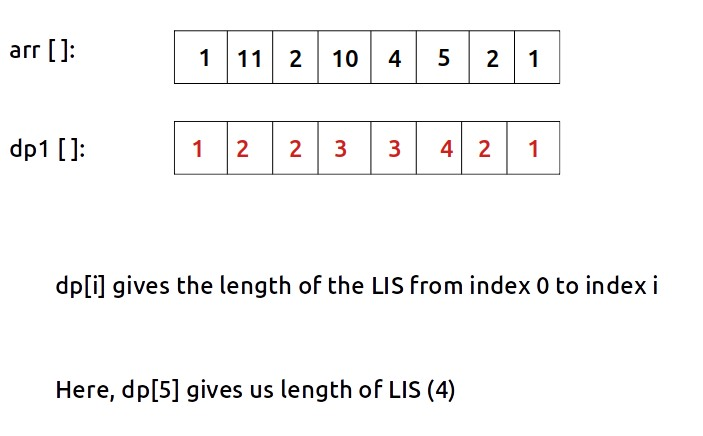Now, after understanding the approach to finding the LIS let us revisit the problem of the longest bitonic subsequence and how it is linked with the problem of LIS.

The below figure breaks general bitonic subsequence into two parts: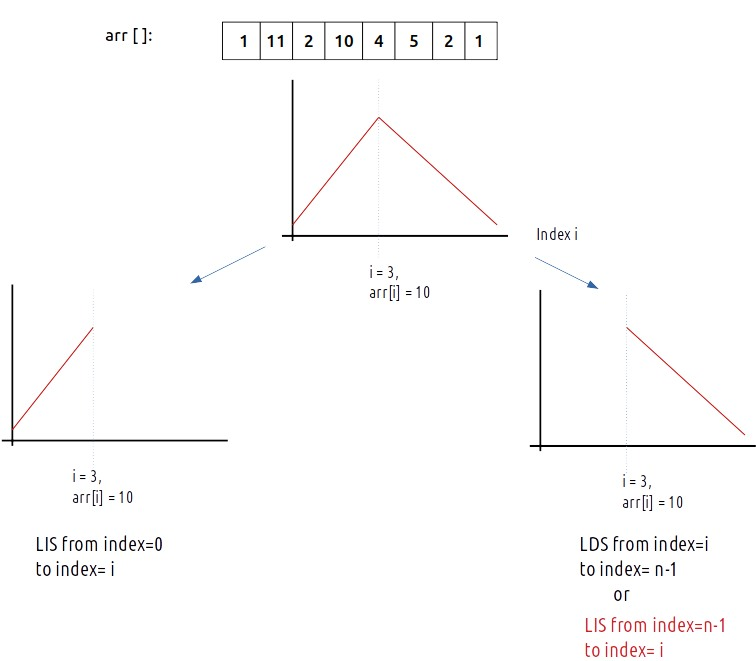As we can see in the second part of the bitonic subsequence, the decreasing part, LDS from index i to index n-1 can also be thought of in terms of LIS from index n-1 to index i. Therefore we can compute this two LIS separately to determine the length of the bitonic subsequence: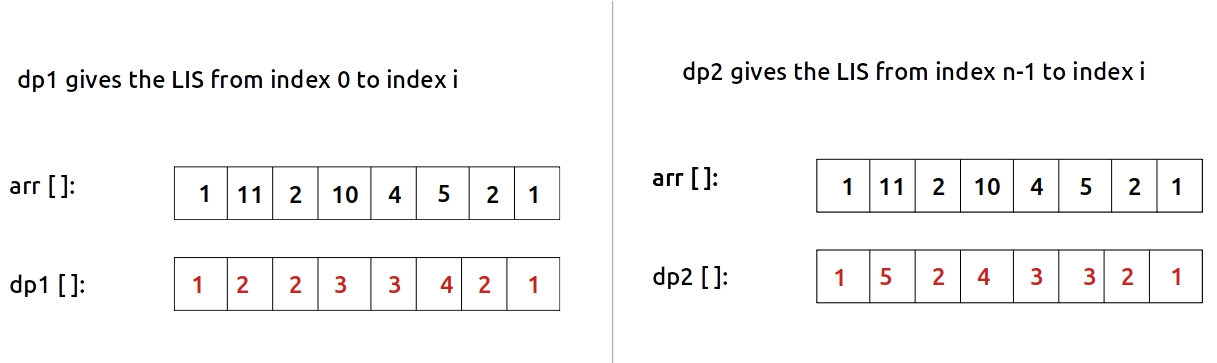Now with these two separate lengths of LIS, we can calculate the length of the longest bitonic subsequence for each index i. Here index i is acting as the pivot point of the points. Therefore the length of the longest bitonic subsequence at pivot [ i ] will be dp1[i] + dp2[i] -1. (Note that we are counting the element at index i twice in both the parts so we need to subtract 1 from the final answer). We will return the maximum value of this addition as the final answer as the length of the longest bitonic subsequence.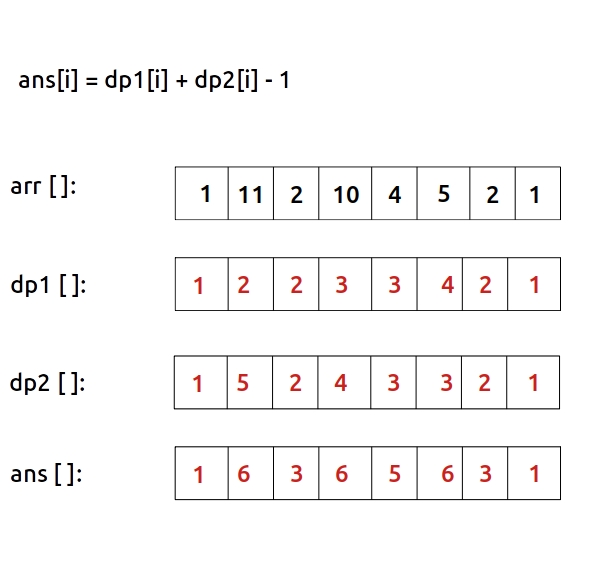Therefore we will return the max in ans array (6) as the final answer.

### Approach:

The algorithm approach is stated as follows:

• Using the approach of the article Printing Longest Increasing subsequence, find the dp1[ ] array, where dp1[i] gives us the length of the LIS from index 0 to index i.
• Modifying the approach slightly, find the dp2[ ] array, where dp2[i] gives us the length of the LIS from index n-1 to index i. To find this opposite direction LIS simply reverses the direction of variables in the nested loops (see the code).
• At last return the answer (the length of the longest bitonic subsequence) as the maximum value of dp1[i] – dp2[i] -1.

Code:

## C++ Code

``````#include <bits/stdc++.h>
using namespace std;

int longestBitonicSequence(vector<int>& arr, int n){

vector<int> dp1(n,1);
vector<int> dp2(n,1);

for(int i=0; i<=n-1; i++){
for(int prev_index = 0; prev_index <=i-1; prev_index ++){

if(arr[prev_index]<arr[i]){
dp1[i] = max(dp1[i], 1 + dp1[prev_index]);
}
}
}

// reverse the direction of nested loops
for(int i=n-1; i>=0; i--){
for(int prev_index = n-1; prev_index >i; prev_index --){

if(arr[prev_index]<arr[i]){
dp2[i] = max(dp2[i], 1 + dp2[prev_index]);
}
}
}

int maxi = -1;

for(int i=0; i<n; i++){
maxi = max(maxi, dp1[i] + dp2[i] - 1);
}

return maxi;

}

int main() {

vector<int> arr = {1, 11, 2, 10, 4, 5, 2, 1};
int n = arr.size();

cout<<"The length of the longest bitonic subsequence is "
<<longestBitonicSequence(arr,n);

return 0;
}
``````

Output:

The length of the longest bitonic subsequence is 6

Time Complexity: O(N*N)

Reason: There are two nested loops that are run twice.

Space Complexity: O(N)

Reason: We are only using two rows of size n.

## Java Code

``````import java.util.*;

class TUF{
static int longestBitonicSequence(int[] arr, int n){

int[] dp1= new int[n];
int[] dp2= new int[n];

Arrays.fill(dp1,1);
Arrays.fill(dp2,1);

for(int i=0; i<=n-1; i++){
for(int prev_index = 0; prev_index <=i-1; prev_index ++){

if(arr[prev_index]<arr[i]){
dp1[i] = Math.max(dp1[i], 1 + dp1[prev_index]);
}
}
}

// reverse the direction of nested loops
for(int i=n-1; i>=0; i--){
for(int prev_index = n-1; prev_index >i; prev_index --){

if(arr[prev_index]<arr[i]){
dp2[i] = Math.max(dp2[i], 1 + dp2[prev_index]);
}
}
}

int maxi = -1;

for(int i=0; i<n; i++){
maxi = Math.max(maxi, dp1[i] + dp2[i] - 1);
}

return maxi;

}
public static void main(String args[]) {

int arr[] = {1, 11, 2, 10, 4, 5, 2, 1};
int n = arr.length;

System.out.println("The length of the longest bitonic subsequence is
"+longestBitonicSequence(arr,n));

}
}
``````

Output:

The length of the longest bitonic subsequence is 6

Time Complexity: O(N*N)

Reason: There are two nested loops that are run twice.

Space Complexity: O(N)

Reason: We are only using two rows of size n.

Special thanks to Anshuman Sharma for contributing to this article on takeUforward. If you also wish to share your knowledge with the takeUforward fam, please check out this articleIf you want to suggest any improvement/correction in this article please mail us at [email protected]